## Dividing a Whole Number by a Proper Fraction

Practice Unlimited Questions

#### How to divide a whole number by a proper fraction?

Dividing a whole number by a proper fraction is the same as multiplying the whole number by the inverse of the proper fraction. Here are the steps:

Step 1:   Change  ÷  to  × .
Step 2:   Invert the fraction.
Step 3:   Simplify the expression.

Example:
Divide 8 by
 4 5
.
8  ÷
 4 5
=  8  ×
 5 4
=  10

#### 1. If a pizza is cut into halves, how many slices do you get?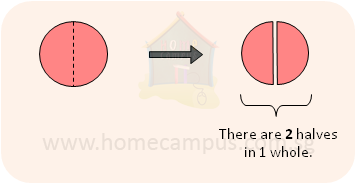1  ÷
 1 2
=  2

You get 2 slices.

#### 2. If 1 whole pizza is cut into one-thirds, how many slices do you get?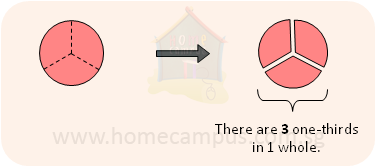1  ÷
 1 3
=  3

You get 3 slices.

#### 3. If 2 pizzas are cut into two-thirds, how many slices do you get?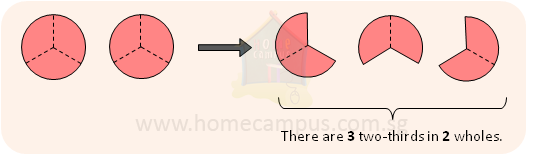2  ÷
 2 3
=  3

You get 3 slices.

#### 4. How many two-thirds are there in 3 wholes?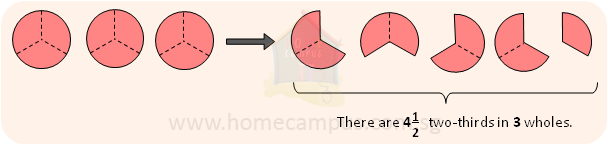3  ÷
 2 3
=
 4 1 2

There are
 4 1 2
two-thirds in 3 wholes.

#### 5. Nina had 2 m of lace that she used for making bows. If she needed     2 7 m of lace for each bow, how many bows did she make?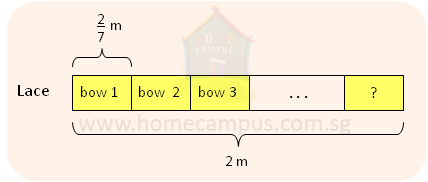2  ÷
 2 7

=  2  ×
 7 2

=  7

#### 6. Mr. Lee buys 25 kg of decorative shells from a wholesale market. He then repacks the shells into small bags of     1 4 kg each. a)   How many bags does he get? b)   If he sells each bag at \$3.50, how much money does he collect from the sale of all bags?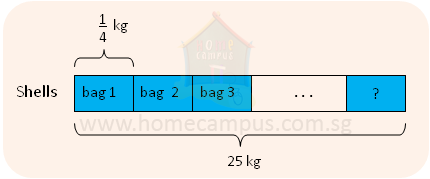a)     25  ÷
 1 4

=  25  ×
 4 1

=  100

He gets 100 bags.

b)     \$3.50  ×  100  =  \$350

He collects \$350.

#### 7. A chemist fills 5 litres of perfume into bottles that have a capacity of     2 11 litre each. a)   What is the greatest number of bottles that he can fill completely? b)   In the end, there is one bottle that is not completely filled. How much perfume does that bottle contain?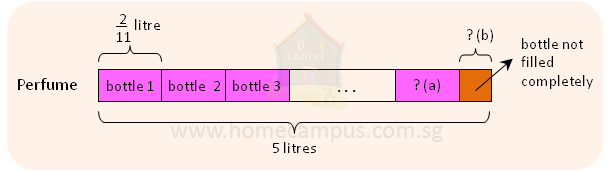a)     5  ÷
 2 11

=  5  ×
 11 2

=
 27 1 2

He can fill 27 bottles completely.

b)     27  ×
 2 11
=
 54 11

There are
 54 11
litres of perfume in the 27 bottles altogether.

5  −
 54 11

=
 55 - 54 11

=
 1 11
litre
The bottle contains
 1 11
litre of perfume.